# Sensors - Synchronous Detection - Part II - EdsCave

Go to content

## Sensors - Synchronous Detection - Part II

Sensors

9 May 2016

How Synchronous Detection Works

In Part 1 of this blog, we introduced the synchronous detection technique as a way to reduce noise in sensing systems. In this installment we will discuss how synchronous detection actually performs its noise-crushing magic.  To do so, we will consider the synchronous detection system as the very conceptualized signal chain shown below.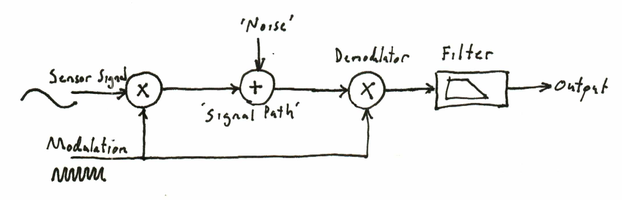In this system, the transducer multiplies its true measured signal by the modulation signal, or carrier.  To do this means that the sensitivity or 'gain' of the sensor is somehow varied by the carrier. For bridge-type sensors (resistive, capacitive, inductive), the sensitivity is proportional to the bias voltage applied to the bridge, so biasing the bridge with the carrier will perform this modulation function. Modulating other types of sensors can require a bit more ingenuity.  What comes out of the bridge is a sinuoidal signal at the carrier frequency where the amplitude and phase are a function of the transducer's measured signal.  Below you can see how the true signal (top-green), combines with the carrier (center-blue) yielding the modulated transducer signal (red-bottom).  While the modulated signal amplitude is proportional to the absolute value of the true signal, the phase is proportional to the sign. When the true signal is positive, the modulated signal will be in phase with the carrier, and when the true signal is negative, the modulated signal will be 180 degrees out of phase with the carrier (opposite in sign).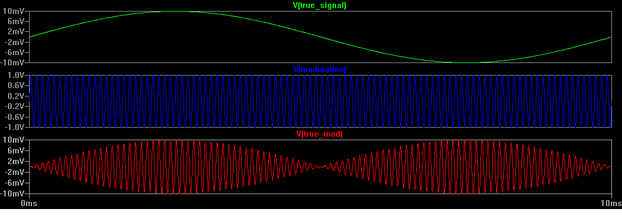Recovering the transducer signal is the reverse process, with post-filtering applied shown below. If we take the modulated transducer signal (green-top) and multiply it again by the carrier (center-blue), we get a result (red-bottom) that has components from both the true signal and carrier. Assuming that the carrier is of much higher frequency than the transducer signals, then it is easy to filter out the carrier component, leaving just the transducer signal (cyan-bottom).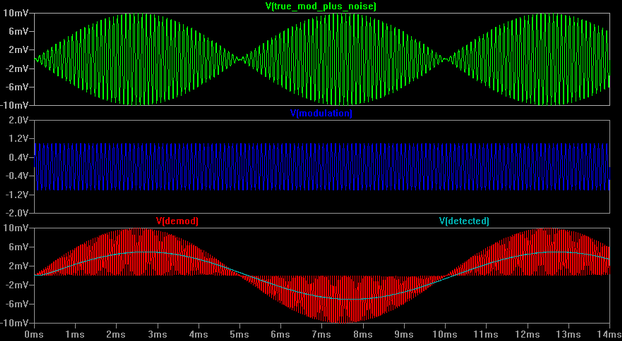The power of synchrounous detection becomes apparent when we apply the demodulation/filter process to something that isn't the modulated transducer signal. In the plots below, the top trace (green) shows an interfering signal comprising both white noise and sinusoidal interference. In this case, there is no modulated transducer signal present.  The sinusoidal interference is set to a frequency (60 Hz) not all that different from that of the transducer signal shown above (100Hz). Because the two frequencies are so close together, trying to remove this interference by filtering would require a fairly sophisticated filter.  Note, however, what happens when the interfering signal is demodulated. Because it is not synchronized with the modulating signal, it ends up being 'chopped' into a roughly equal number of positive and negative parts at the modulating frequency (red-bottom). This is in contrast the case where the modulated transducer signal was demodulated and the 'pieces' got distributed positive or negative so as to represent the true transducer signal. When the demodulated interference signal is filtered, it results in near-zero output (bottom trace - cyan)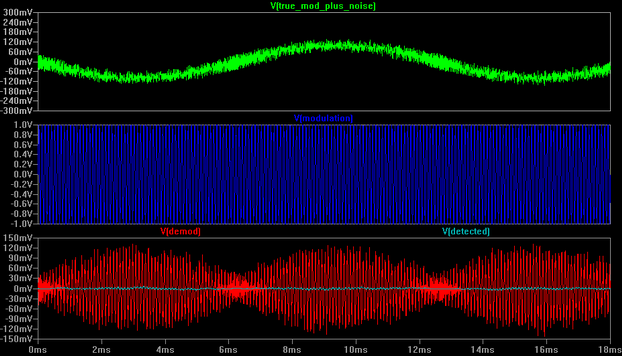The demodulation and filtering process is very similar to finding the statistical covariance between the modulated transducer signal and the carrier.  The only signals that would be expected to have a high covariance (either postive or negative) with the carrier are those that have very similar frequency and phase characteristics.  Using a carrier with significantly higher frequency than that of the transducer signal allows one to effectively take more 'samples' for the covariance (longer filtering time) so as to get more significant results.

Another way to understand how synchronous detection works is through its frequency-domain behavior.  This approach is significantly more abstract than looking at how time-domain signals are processed, but yields better insights into how the technique works.  In the frequency domain, signal energy (Y-axis) is plotted against the signal's frequency (x-axis). This plot show the 'frequency spectrum' of the signal.  A sinusoidal waveform is the most fundamental type of signal and appears as a single spike at a given frequency.  More complex signals, which contain a mixture of frequency components, such as those coming from a transducer or interference will often appear as areas. The spectrum plot below shows a sensor signal (blue) containing a mix of frequencies starting at zero frequency (DC or constant value) and tapering off as the frequency increases. It also shows an interference signal (red) that is a mixure of frequencies centered around a non-zero value.  This combination of signals is what you would see if you measured the sensor signal with a traditional amplifer/filter system. The challenge would be devising a filter that was sufficiently selective so that it blocks the noise while passing the sensor signal.The effect of modulating the sensor signal is to shift it upward in frequency by the carrier frequency (Fmod - green spike shown below).  A side effect of this is that a mirror image of the sensor signal's spectrum is created beneath Fmod.  Notice that the noise spectrum stays where it was originally becuause it is not being modulated, but is just being added in to the sensor signal after it has been up-shifted by the modulation.Now if we demodulate the above spectrum using the same carrier frequency,  several things happen:

• The previously modulated transducer signal is shifted back down so that it at DC. Additionally, (but not shown), a copy of the transducer spectrum is created  that is centered at 2 x Fmod (twice the modulation frequency).

• The noise signal is upshifted by Fmod. Also, a second copy is created that is symmetrically mirrored around Fmod, but on the lower side.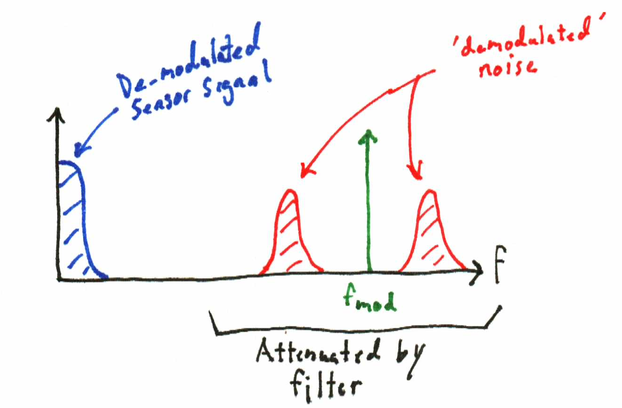If Fmod is significantly higher that the transducer signals, then it shifts any interference in proximity to the transducer signals far away from the transducer signals. Since it has been moved away from the transducer signals, the interference can then be easily filtered out.

But what about the case where the interference is close to the carrier frequency? This does pose a problem. If the interference is at the same frequency and phase as the modulation signal, it will be detected along with the signal. One big advantage of synchronous detection is that within wide limits, you get to pick your modulating frequency.  For example, in the US 60Hz is the standard power-line frequency and consequently there is a lot of interference in the environment at 60Hz and its multiples. If you choose to modulate your sensor at 60Hz this could cause significant problems significant. On the other hand, however, you might choose to modulate at some other frequency, such as 80Hz which will reject the 60Hz interference.  Synchronous detection is not a magic bullet, but does require one to consider the application requirements to sucessfully employ it.

In the final part of this blog we will discuss some techniques for practical implementation.